## Search# How to insert and edit equations

Flash guide

1. In the Editor at plickers.com, navigate to your question prompt or answer choice
2. Select the Insert Equationicon in the main toolbar at the top
3. Enter your equation by using LaTeX notation or the buttons provided
4. Click the blue Insert Equation button

Our Equation Editor provides an opportunity to write math and science notation (with LaTeX support) for equations both simple and complex. In this article we take you through how to use it to insert new equations, as well as edit existing ones.

##### Below you can see what the Equation Editor looks like:## Insert an Equation

You'll arrive at your Plickers homepage.

2. ## Access the Editor at plickers.com

You can do so by either signing in to plickers.com and selecting New Set, or by double clicking on the title of the content item you want to edit. To find out more, check out our Editor article linked here.

3. ## Choose where you want to insert your equation

You can do this by clicking into the text box for either the question prompt or any of the choices.

Tip

For questions, we would advise inserting an equation into a fresh line of your prompt, to make things as clear as possible for your students.

4. ## Click on the Insert Equation iconin the main toolbar at the top5. ## Use the Equation Editor to create an equation

You can type in letters and numbers as normal into the Equation Editor, but there are two possible ways to enter symbols:

• If you're experienced with using LaTeX, you may be comfortable typing out what you need within the Equation Editor.

To enter a symbol, simply hit the \ key, type the name of the symbol, then hit the spacebar. For example:

• if you type \sqrt and hit the spacebar, you'll see• if you type \pi and hit the spacebar, you'll seeHere's a gif to demonstrate: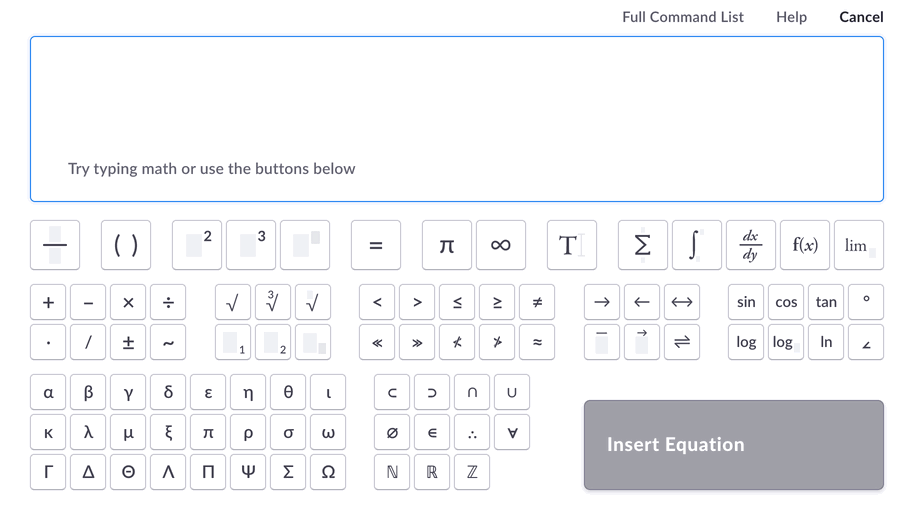Tip

Use the arrow keys on your keyboard to move between data entry points. For example, moving from inside to outside the square root symbol to continue writing the equation.

• It's also possible to use the mouse to insert symbols by clicking the buttons provided (like you might do on a calculator for instance).

The gif below shows how to write the same formula as above, but by using the mouse to insert the integral, square root and pi symbols.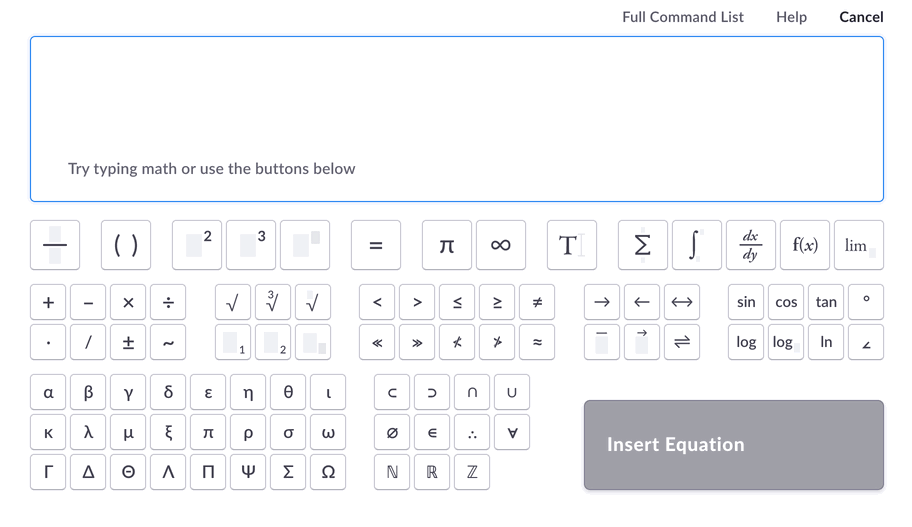Tip

Instead of using the arrow keys to move between data entry points, it might be more convenient simply to click the area where you want to add text (as shown in the gif above).

6. ## Click on the blue 'Insert' button

This will insert the equation into the area of the canvas that you previously selected (either the question prompt or one of the choices).

In our example, we previously chose to insert the equation into the question prompt, so this is what it looks like when we press the button: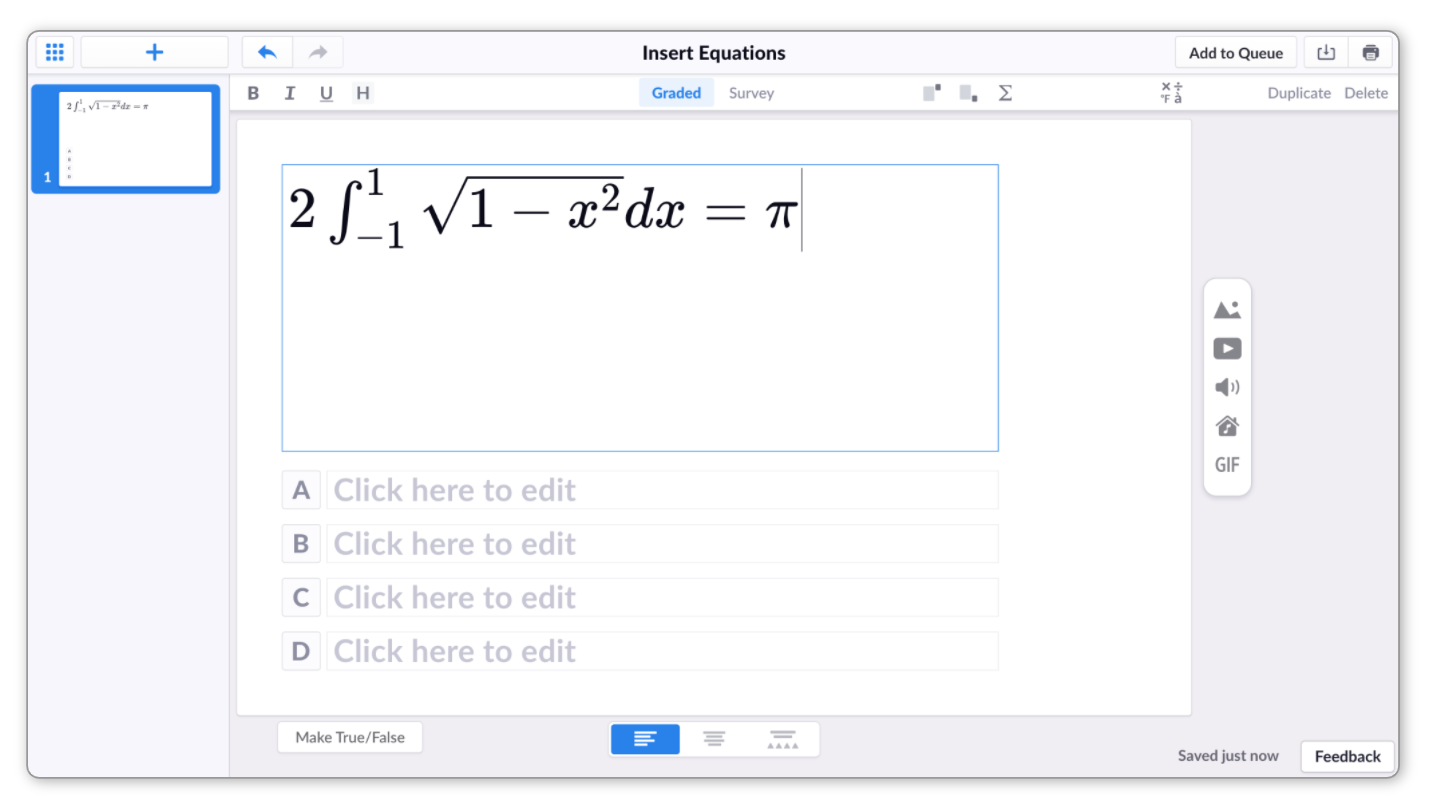It's possible to copy equations between questions and answer choices. Check out the relevant section below to find out more.

## Edit an Equation

Once you've inserted an equation, it's very easy to edit it again; all you really need to do is click on it.

1. ## Navigate to the equation you'd like to edit

When you hover your cursor over the equation you'll notice a pale blue background appear, which indicates the extent of that equation.2. ## Click on the equation

When you see the pale blue background appear, click your mouse and you'll be taken to the Equation Editor.

Use the Equation Editor to make your changes. There's information on how to do this available in the Insert an Equation section above.

4. ## Click the blue 'Save Changes' button

When you're happy with your edits, select the blue Save Changes button, and you'll see the changes reflected on the canvas.

##### Click on the toggle box below to see a gif demonstrating what this overall process looks like:
•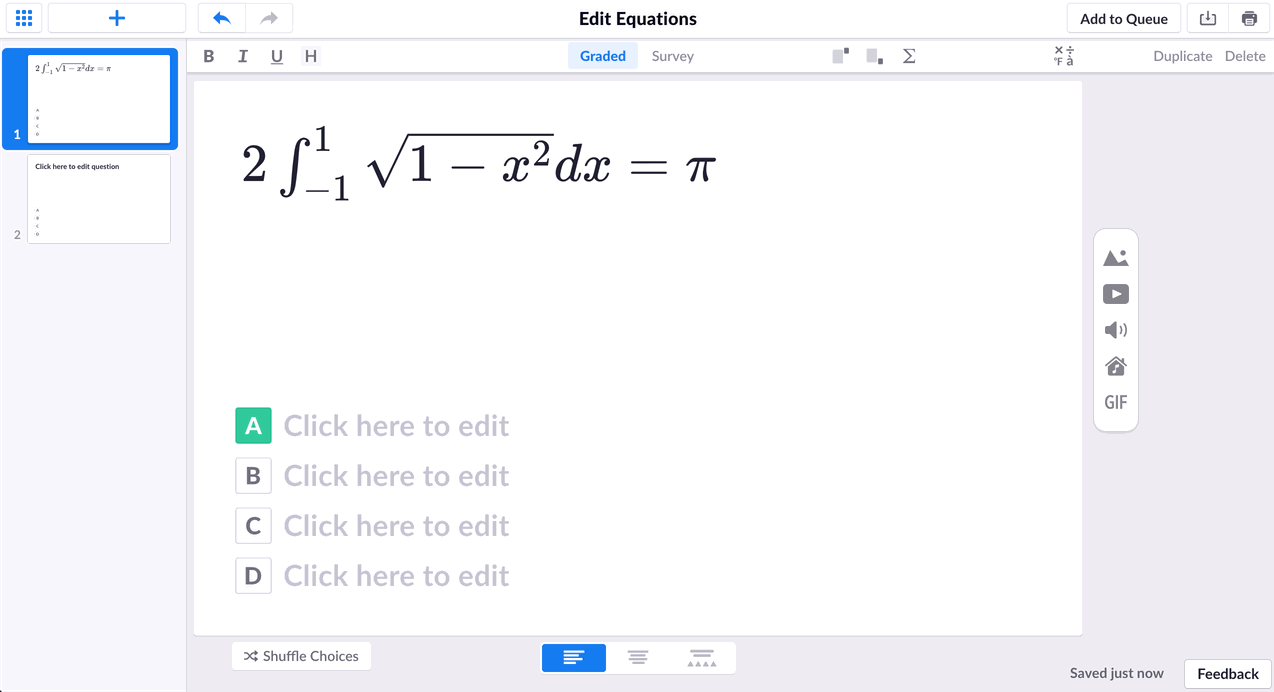Tip

If you prefer navigating with your keyboard, you can also use your arrow keys to select the equation in the question canvas and then hit enter to edit.

## Move an Equation

There might come a time when you need to copy an equation into an answer choice or another question. There are two ways to do this:

• This method requires the use of the Equation Editor both when you copy the equation, and when you paste it. It's also worth noting that this is the way to go if you need to copy only a section of an equation.

Here's a gif to help visualize the process of copying text from one Equation Editor to another. The full instructions follow underneath.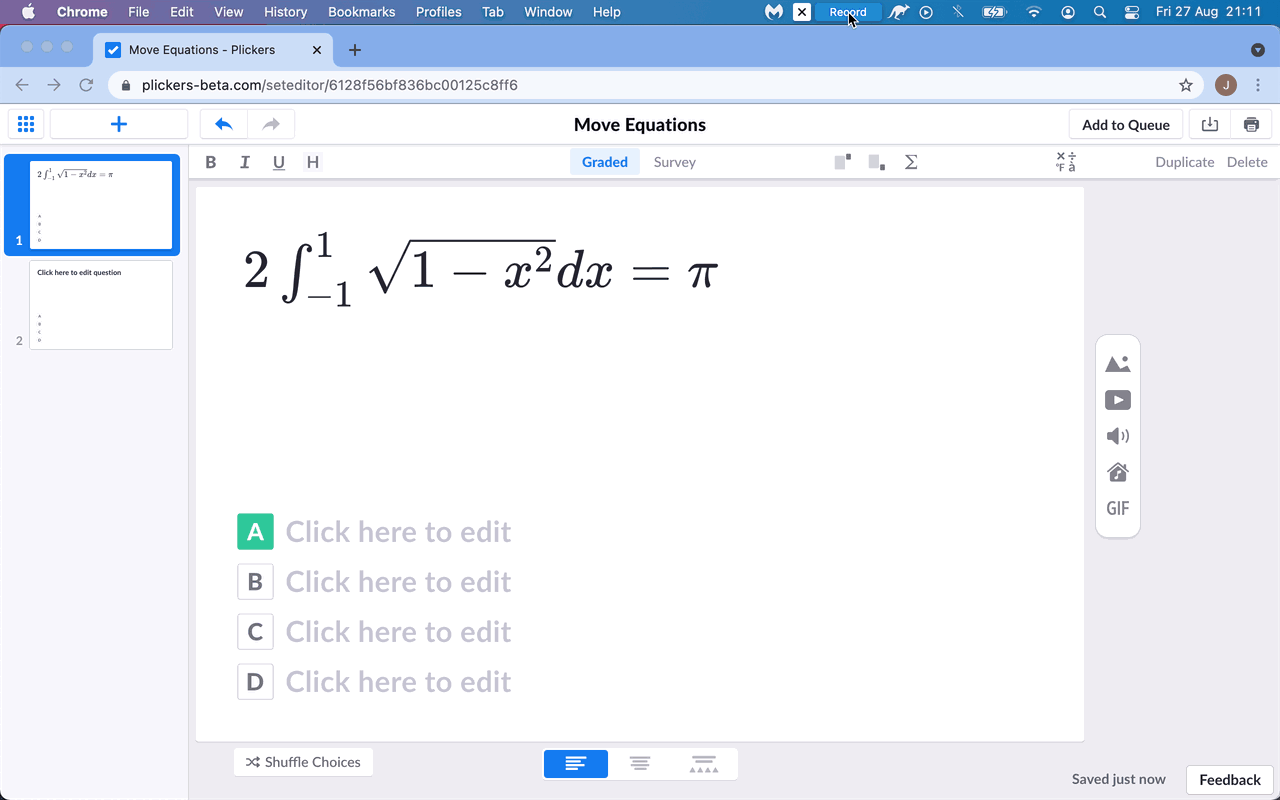1. ## Navigate to the equation you'd like to copy

When you hover your cursor over the equation you'll notice a pale blue background appear, which indicates the extent of that equation.2. ## Click on the equation

You'll be taken to the Equation Editor.

3. ## Highlight your equation in the Equation Editor

You can use your mouse, or press ctrl/cmd + A on your keyboard.

4. ## Copy the equation

You can either select the main Edit menu and select 'Copy', or press ctrl/cmd + C on your keyboard.

5. ## Click 'Cancel' in the top right

Or you can simply hit the 'Escape' key.

6. ## Navigate to the destination slide for your equation

Then click into the relevant question or answer choice text box.

7. ## Select the Insert Equation iconin the top toolbar

You can do this by selecting the main Edit menu and selecting 'Paste', or simply by pressing ctrl/cmd + V on your keyboard.

9. ## Select the blue 'Insert Equation' button

Your equation will be inserted into its new location.

• This method allows you to copy and paste equations without entering the Equation Editor.

Here's a gif to demonstrate the process (the full instructions follow underneath).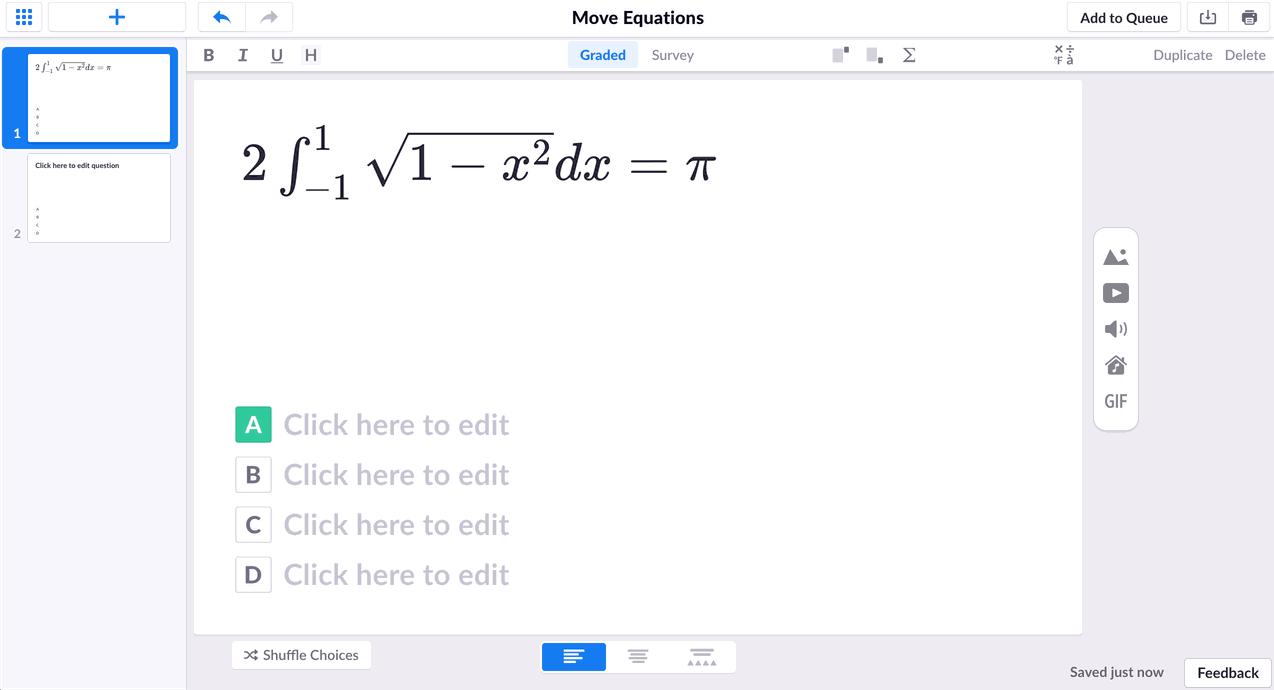2. ## Highlight the full equation

You can use your mouse to do this, or click into the text box and press ctrl/cmd + A to select all.

Note the difference between the pale blue background that shows when your mouse is hovering over an equation, and the darker blue highlight that signifies an equation is selected.

When copying an equation, you'll want to ensure you see the darker highlight. The images below show the difference to look out for.3. ## Copy the equation

You can either right-click on the equation and select 'Copy', or press ctrl/cmd + C on your keyboard.

4. ## Navigate to the destination slide for your equation

Then click into the relevant question or answer choice text box.

You can do this by right-clicking and selecting 'Paste', or simply by pressing ctrl/cmd + V on your keyboard.

Note

It's not possible to mix the two methods above, i.e. copying an equation from the Equation Editor and pasting it straight into a text box on the canvas, or vice versa.

Thanks for reading through our guide on using equations within your Plickers content. If you have any follow-up questions, please feel free to contact our friendly support team at support@plickers.com